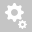##Recently viewed tickets# [DESIGN]     How is effective length factor calculated?

A: In midas Gen, effective length factor could either be given manually or the program auto computes based on the frame definition

Auto Calculate Effective Length factor:
For the program to automatically consider effective length factor, from the main menu select Design > General Design parameter > Definition of frame. Check on ‘Auto Calculate Effective Length Factors’ for the program to internally calculate the effective length factor.The auto-calculated effective length is based on the procedure briefed as follows:
Let us consider the ‘Member A’ as shown in Figure 1

1. Calculate stiffness, S (=EI/L), of the members which are connected to the ‘Member A’. If the joint of the flexural member is fixed or hinged as shown in the figure 2 below, the stiffness, S, is modified as indicated.where,
E: Modulus of elasticity
I: Moment of inertia of section
L: Span length of flexural member measured from center to center of joints

2. Calculate Ψi and Ψj. If the end of the compression member is fixed or hinged, Ψ is taken as 1 or 10 respectively, If the compression member is not connected to any flexural member, Ψ is taken as 1000. Here Ψ, is ratio of Σ(EI/lc) of compression members (Columns) to Σ(EI/l) of flexural members (Beams) in a plane at either i-end or j-end of the compression member.

3. Calculate the solution, X, in the stability equation below.
Braced / Non-sway framesUn braced / sway frameswhere , Σ(EI/lc) is summation of stiffness of columns at a joint ( Either i-end or j-end)
Σ(EI/lb) is summation of stiffness of beams connected at same joint

4. Calculate the effective length factor,

Reference: "Steel structures" (1982), Ballio and Mazzolani

User defined Effective Length factor:

From the main menu select Design > General Design parameter > Effective Length Factor. Ky, Kz, Kt: :Effective Length Factors for the unbraced lengths about strong axis, weak axis and for torsion
If the effective buckling length factor is repeatedly entered for the same member, the following procedure is used to set the applicable value :
1. The effective buckling length factor last entered by the user

2. In case when the Factors are not entered, and If 'Auto Calculate K-Factor' is selected in Definition of Frame, the program automatically calculates Ky and Kz.

3.If None of the above, the program applies the default value of 1.0 to Ky, Kz and Kt.

The entered effective buckling length factors can be reviewed in the data table arranged in the order of element numbers. The user may Modify/Add and Delete items in the data table.Creation date: 8/18/2017 11:57 AM ()      Updated: 9/5/2017 8:26 AM ()